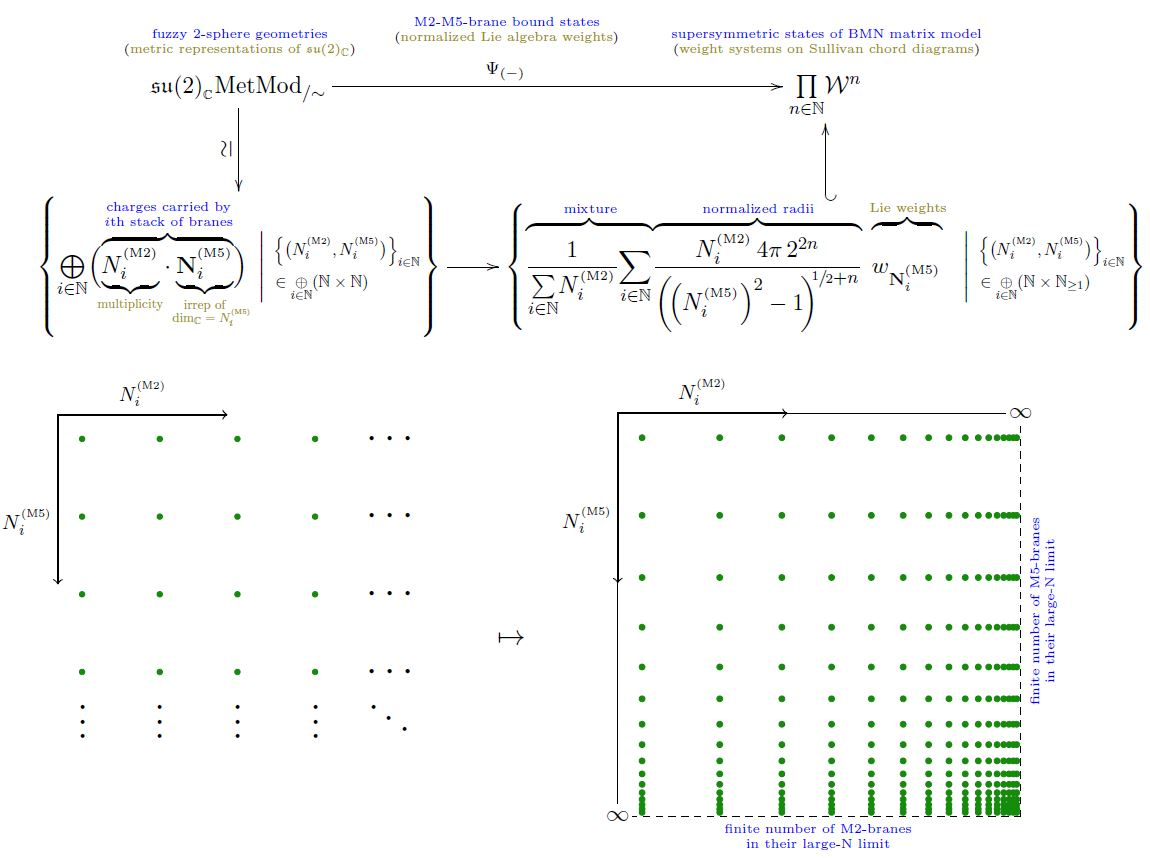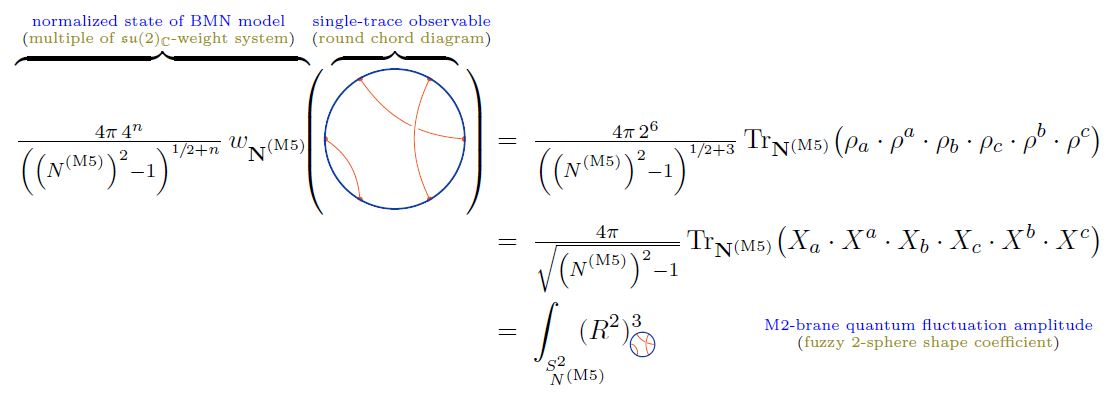# nLab M2-M5 brane bound states in the BMN matrix model -- subsection

M2-M5 brane bound states in the BMN matrix model

### M2-M5 brane bound states in the BMN matrix model

There is the suggestion (MSJVR 02, checked in AIST 17a, AIST 17b) that, in the BMN matrix model, supersymmetric M2-M5-brane bound states are identified with isomorphism classes of certain “limit sequences” of longitudinal-light cone-constant $N \times N$-matrix-fields constituting finite-dimensional complex Lie algebra representations of su(2).

Concretely, if

$\underset{ i }{\oplus} \big( N^{(M2)}_i \cdot \mathbf{N}^{(M5)}_i \big) \;\;\in\;\; \mathfrak{su}(2)_{\mathbb{C}}Rep^{fin}$

denotes the representation containing

of the

• $N^{(M5)}_i$-dimensional irrep $\mathbf{N}^{(M5)}_i \in \mathfrak{su}(2)_{\mathbb{C}}Rep$

(for $\{N^{(M2)}_i, N^{(M5)}_i\}_{i} \in (\mathbb{N} \times \mathbb{N})^I$ some finitely indexed set of pairs of natural numbers)

with total dimension

$N \;\coloneqq\; dim \big( \underset{ i }{\oplus} \big( N^{(M2)}_i \cdot \mathbf{N}^{(M5)}_i \big) \big)$

then:

1. a configuration of a finite number of stacks of coincident M5-branes corresponds to a sequence of such representations for which

1. $N^{(M2)}_i \to \infty$ (this being the relevant large N limit)

2. for fixed $N^{(M5)}_i$ (being the number of M5-branes in the $i$th stack)

3. and fixed ratios $N^{(M2)}_i/N$ (being the charge/light-cone momentum carried by the $i$th stack);

2. an M2-brane configuration corresponds to a sequence of such representations for which

1. $N^{(M5)}_i \to \infty$ (this being the relevant large N limit)

2. for fixed $N^{(M2)}_i$ (being the number of M2-brane in the $i$th stack)

3. and fixed ratios $N^{(M5)}_i/N$ (being the charge/light-cone momentum carried by the $i$th stack)

for all $i \in I$.

Hence, by extension, any other sequence of finite-dimensional $\mathfrak{su}(2)$-representations is a kind of mixture of these two cases, interpreted as an M2-M5 brane bound state of sorts.

#### Formalization via weight systems on chord diagrams

To make this precise, let

$\mathfrak{su}(2)_{\mathbb{C}} MetMod_{/\sim} \;\in\; Set$

be the set of isomorphism classes of complex metric Lie representations (hence finite-dimensional representations) of su(2) (hence of the special linear Lie algebra $\mathfrak{sl}(2,C)$) and write

$Span \big( \mathfrak{su}(2)_{\mathbb{C}} MetMod_{/\sim} \big) \;\in\; Vect_{\mathbb{C}}$

Finally, write

$\array{ Span \big( \mathfrak{su}(2)_{\mathbb{C}}MetMod_{/\sim} \big) & \longrightarrow & \big( \mathcal{W}^{{}^{s}} \big)^{deg} \\ c_1 \cdot V_1 + c_2 \cdot V_2 &\mapsto& c_1 \cdot w_{V_1} + c_2 \cdot w_{V_2} }$

for the linear map which sends a formal linear combination of representations to the weight system on Sullivan chord diagrams with $deg \in \mathbb{N}$ chords which is given by tracing in the given representation.

Then a M2-M5-brane bound state as in the traditional discussion above, but now formalized as an su(2)-weight system

$\Psi_{ \left\{ N^{(M2)}_i, N^{(M5)}_i \right\}_{i} } \;\in\; \underset{ deg \in \mathbb{N} }{\prod} \big( \mathcal{W}^{{}^{\mathrm{s}}} \big)^{deg}$

hence a weight system horizontal chord diagrams closed to Sullivan chord diagrams, these now being the multi-trace observables on these) is

(1)$\Psi_{ \left\{ N^{(M2)}_i, N^{(M5)}_i \right\}_{i} } \;\coloneqq\; \tfrac{ 4 \pi \, 2^{2\,deg} }{ \sum_i N^{(M2)}_i } \underset{i}{\sum} \left( N^{(M2)}_i \cdot \tfrac{1}{ \left( \sqrt{ \left( N^{(M5)}_i \right)^2 - 1 } \right)^{1 + 2 deg} } \mathbf{N}^{(M5)}_i \right)$(from Sati-Schreiber 19c)

Normalization and large $N$ limit. The first power of the square root in (1) reflects the volume measure on the fuzzy 2-sphere (by the formula here), while the power of $2\,deg$ (which is the number of operators in the multi-trace observable evaluating the weight system) gives the normalization (here) of the functions on the fuzzy 2-sphere.

Hence this normalization is such that the single-trace observables among the multi-trace observables, hence those which come from round chord diagrams, coincide on those M2-M5 brane bound states $\Psi_{ \left\{ N^{(M2)}_i, N^{(M5)}_i \right\}_{i} }$ for which $N^{(M2)}_i = \delta_i^{i_0} N^{(M2)}$, hence those which have a single constitutent fuzzy 2-sphere, with the shape observables on single fuzzy 2-spheres discussed here:(from Sati-Schreiber 19c)

Therefore, with this normalization, the limits $N^{(M2)} \to \infty$ and $N^{(M5)} \to \infty$ of (1) should exist in weight systems. The former trivially so, the latter by the usual convergence of the fuzzy 2-sphere to the round 2-sphere in the large N limit.

Notice that the multi trace observables on these states only see the relative radii of the constitutent fuzzy 2-spheres: If $N^{(M2)}_i = \delta_i^{i_0} N^{(M2)}$ then the $N^{(M2)}$-dependence of (1) cancels out, reflecting the fact that then there is only a single constituent 2-sphere of which the observable sees only the radius fluctuations, not the absolute radius (proportional to $N^{(M2)}$).

Last revised on July 7, 2023 at 15:46:17. See the history of this page for a list of all contributions to it.